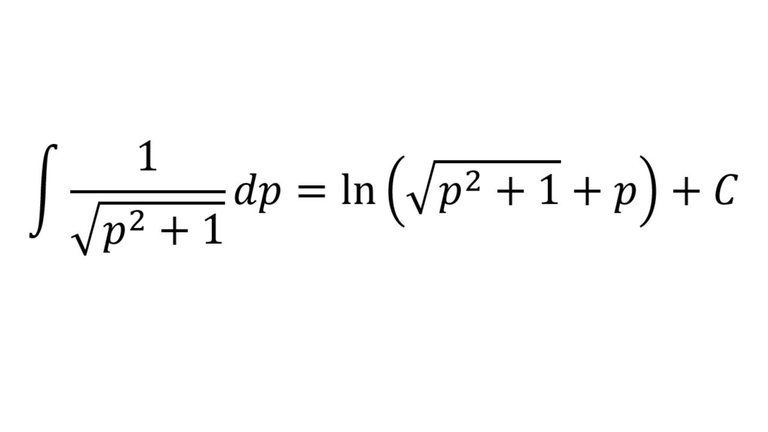# Integrals Example: Trigonometric Substitution: Integral from Catenary Proof

in Threespeaklast month (edited)In this video I go over an example on using trigonometric substitution to evaluate integrals. This example is the same one in my earlier catenary proof video, but which I used an online integral calculator to save time in the derivation. The integral is of the function 1/sqrt(p2 + 1) and I show how it is pretty straight forward to apply the trig substitution p = tan(u) because then we can use trigonometry identities to remove the square root in the integrand. The solution to the integral involves an absolute value, but I also show how in this particular example I show how the function inside the absolute value is always greater than 0, and thus we can remove the absolute value sign! This is a great example on applying the trigonometric substitution as well as in understanding how absolute value functions can be simplified, so make sure to watch this video!

Download the notes in my video: https://1drv.ms/b/s!As32ynv0LoaIhvtFA2MyUYYYRCZZJQ

View video notes on the Hive blockchain: https://peakd.com/mathematics/@mes/video-notes-integrals-example-trigonometric-substitution-integral-from-catenary-proof

Related Videos:

Hyperbolic Functions: Catenary: Formula and Proof: https://youtu.be/EYb1p9r1fnM
Trigonometric Substitution for Integrals: http://youtu.be/2pWvGXwtVJo
Trigonometry: Derivative of sec(x): Proof: http://youtu.be/0sO0TehqMOw
Improper Integrals: Example 6: sec(x): https://youtu.be/yHnv2w9OVWk
Trigonometry Identity: tan2(x) + 1 = sec2(x): https://youtu.be/5i3NZ_xip7I .

SUBSCRIBE via EMAIL: https://mes.fm/subscribe

DONATE! ʕ •ᴥ•ʔ https://mes.fm/donate

Like, Subscribe, Favorite, and Comment Below!

Follow us on:

MES Truth: https://mes.fm/truth
Official Website: https://MES.fm
Hive: https://peakd.com/@mes
Gab: https://gab.ai/matheasysolutions
Minds: https://minds.com/matheasysolutions
Twitter: https://twitter.com/MathEasySolns
Facebook: https://fb.com/MathEasySolutions
LinkedIn: https://mes.fm/linkedin
Pinterest: https://pinterest.com/MathEasySolns
Instagram: https://instagram.com/MathEasySolutions
Email me: [email protected]

Free Calculators: https://mes.fm/calculators

BMI Calculator: https://bmicalculator.mes.fm
Grade Calculator: https://gradecalculator.mes.fm
Mortgage Calculator: https://mortgagecalculator.mes.fm
Percentage Calculator: https://percentagecalculator.mes.fm

Free Online Tools: https://mes.fm/tools

iPhone and Android Apps: https://mes.fm/mobile-apps

▶️ 3Speak

Sort:

it is always amazing to see you solve math problems